# Between two bus stops

Wanda lives between two bus stops at three-eighths of their distance. He started the house today and found that whether he was running to one or the other stop, he would have arrived at the bus stop. The average bus speed is 60 km/h.

What is the average speed of Wanda running today?

Result

v =  15 km/h

#### Solution:

$s_{ 1 } = \dfrac{ 3 }{ 8 } s \ \\ s_{ 2 } = s-s_{ 1 } = \dfrac{ 5 }{ 8 } s \ \\ \ \\ v_a = 60 \ km/h \ \\ \ \\ s_{ 1 } = v \cdot \ t_{ 1 } \ \\ s_{ 2 } = v \cdot \ t_{ 2 } \ \\ \ \\ s_{ 3 } = v_a \cdot \ t_{ 1 } \ \\ s_{ 3 }+s = v_a \cdot \ t_{ 2 } \ \\ v_a \cdot \ t_{ 1 } + s = v_a \cdot \ t_{ 2 } \ \\ \ \\ v_a \cdot \ s_{ 1 }/v + s = v_a \cdot \ s_{ 2 }/v \ \\ v_a \cdot \ \dfrac{ 3 }{ 8 } \cdot \ s/v + s = v_a \cdot \ \dfrac{ 5 }{ 8 } \cdot \ s/v \ \\ v_a \cdot \ \dfrac{ 3 }{ 8 } /v + 1 = v_a \cdot \ \dfrac{ 5 }{ 8 } /v \ \\ v_a \cdot \ \dfrac{ 3 }{ 8 } + v = v_a \cdot \ \dfrac{ 5 }{ 8 } \ \\ \ \\ v = v_a \cdot \ \dfrac{ 5 }{ 8 } - v_a \cdot \ \dfrac{ 3 }{ 8 } \ \\ \ \\ v = v_a \cdot \ ( \dfrac{ 5 }{ 8 } - \dfrac{ 3 }{ 8 } ) = 60 \cdot \ ( \dfrac{ 5 }{ 8 } - \dfrac{ 3 }{ 8 } ) = 15 = 15 \ \text { km/h }$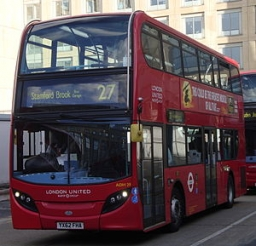Our examples were largely sent or created by pupils and students themselves. Therefore, we would be pleased if you could send us any errors you found, spelling mistakes, or rephasing the example. Thank you!

Leave us a comment of this math problem and its solution (i.e. if it is still somewhat unclear...):

Showing 1 comment:Matematik
I bow to the author of the solution, it is brilliant :)#### Following knowledge from mathematics are needed to solve this word math problem:

Looking for help with calculating arithmetic mean? Looking for help with calculating harmonic mean? Looking for a statistical calculator? Do you have a linear equation or system of equations and looking for its solution? Or do you have quadratic equation?

## Next similar math problems:

1. Father and sonWhen I was 11, my father was 35 years old. Today, my father has three times more years than me. How old is she?
2. Journey to school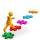On the way to school I went steady step. In the first half of the journey, I counted every second step, in the second half every third. How many steps I do when I went to school if I counted double step are 250 more than three steps?
3. Klara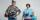Klara and Jitka went on a hiking trip at 13 o'clock at speed 5km/h. At 14 o'clock, Tomas ride on the bike at an average speed of 28 km/h. How many hours and at what distance from the beginning of the road Tomáš caught the two girls?
4. Pedestrian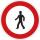Pedestrian came started at 8h in the morning with speed 4.4 km/h. At half-past eleven cyclist started at 26 km/h same way. How many minutes take cyclist to catch up pedestrian?
5. Cenda and Pepa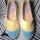Cenda and Pepa went to the event. Cenda started alone. Canda started after him for 20 minutes. How long took Cenda to catch him? Cenda traveling at 15 km/h, and Pepa traveling at 25 km/h.
6. Cars 6At 9:00 am two cars started from the same town and traveled at a rate of 35 miles per hour and the other car traveled at a rate of 40 miles per hour. After how many hours will the cars be 30 miles apart?
7. Cyclist vs car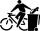Cyclist rode out of the city at 18 km/h. 1 hour 30 minutes behind him started car and caught up with the cyclist in 50 minutes. How fast was driving the car? Where (what kilometer) from the city car overtook a cyclist?
8. Where and when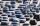The truck left Kremnica at 11:00 h at a speed of 60km/h. At 12:30 h, the passenger car started at an average speed of 80km/h. How many kilometers from Kremnica will the passenger car reach truck, and when?
9. Six yearsIn six years Jan will be twice as old as he was six years ago. How old is he?
10. Average speedThe truck drove 1/2 of the way on the highway at 80km/h. The other half of the way 20km/h. Calculate the average speed
11. Drive to NJ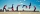Ed drove to New Jersey at 30mph. He drove back home in 3 hours at 50 mph. How many hours did it take Ed to drive to New Jersey?
12. Two shipsTwo ships sailed from Venice - a cargo speed of 10 knots and a ferry speed of 18 knots. At what time will the ferry catch up with the cargo ship if it sailed 10 hours later?
13. Round-tripA woman works at a law firm in city A which is about 50 miles from city B. She must go to the law library in city B to get a document. Find how long it takes her to drive​ round-trip if she averages 40 mph.
14. Coach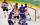Average age of 24 players and a coach of one team is 24 years. The average age of players without a coach is 23 years. How old is the coach?
15. StormSo far, a storm has traveled 35 miles in 1/2 hour in direction straight to observer. If it is currently 5:00 p. M. And the storm is 105 miles away from you, at what time will the storm reach you? Explain how you solved the problem.
16. Trees avgThe worker planted 96 trees on Monday, 120 on Tuesday and 61 trees on Wednesday. How many trees did he plant on Thursday if he averaged 105 trees per day?
17. Greg and BillGreg is 18 years old. He is 6 less than 4 times Bill's age. How old is Bill?